3-D VisualizationDefault Aspect Ratio Selection

There are two key elements to MATLAB default behavior -- normalizing the axes size to the window size and stretch-to-fill.

The axes `Position` property specifies the location and dimensions of the axes. The third and fourth elements of the `Position` vector (`width` and `height`) define a rectangle in which MATLAB draws the axes (indicated by the dotted line in the following pictures). MATLAB stretches the axes to fill this rectangle.

The default value for the axes `Units` property is `normalized` to the parent figure dimensions. This means the shape of the figure window determines the shape of the position rectangle. As you change the size of the window, MATLAB reshapes the position rectangle to fit it.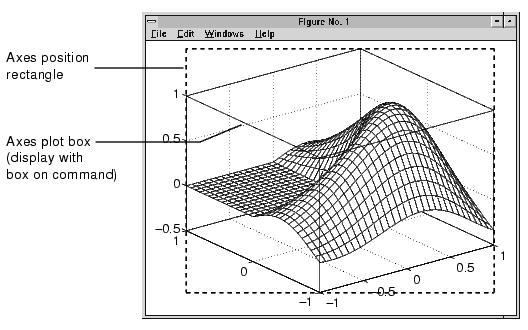The view is the 2-D projection of the plot box onto the screen.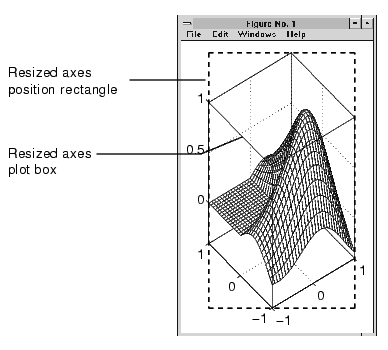As you can see, reshaping the axes to fit into the figure window can change the aspect ratio of the graph. MATLAB applies stretch-to-fill so the axes fill the position rectangle and in the process can distort the shape. This is generally desirable for graphs of numeric data, but not for displaying objects realistically.

Example -- MATLAB Defaults

MATLAB surface plots are well suited for visualizing mathematical functions of two variables. For example, to display a mesh plot of the function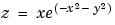evaluated over the range -2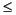x2, -4y4, use the statements

• ```[X`,`Y]` `=` `meshgrid([-2:.15:2],[-4:.3:4]);
Z` `=` `X.*exp(-X.^2` `-` `Y.^2);
mesh(X,Y,Z)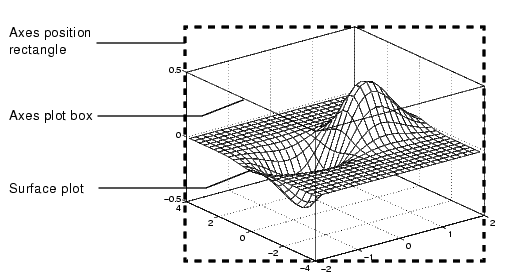```

The MATLAB default property values are designed to

• Select axis limits to span the range of the data (`XLimMode`, `YLimMode`, and `ZLimMode` are set to `auto`).
• Provide the highest resolution in the available space by setting the scale of each axis independently (`DataAspectRatioMode` and the `PlotBoxAspectRatioMode` are set to `auto`).
• Draw axes that fit the position rectangle by adjusting the `CameraViewAngle` and then stretch-to-fill the axes if necessary.Axes Aspect Ratio Properties Overriding Stretch-to-Fill© 1994-2005 The MathWorks, Inc.